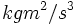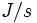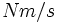# Power

MLT dimensions?$ML^2T^{-3}$: MLT;1;2;-3
SI units?$W$ (Watt), equal to$kgm^2/s^3$ or$J/s$ (Joules per second) or$Nm/s$ (Newton-meters per second)